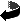# Manifolds with small Dirac eigenvalues are nilmanifolds by Bernd Ammann, Chad Sprouse

Manifolds with small Dirac eigenvalues are nilmanifolds (.dvi, .ps, .ps.gz or .pdf)
Ann. Glob. Anal. Geom. 31, 409-425 (2007)
DOI 10.1007/s10455-006-9048-2

### Abstract

Consider the class of n-dimensional Riemannian spin manifolds with bounded sectional curvatures and diameter, and almost non-negative scalar curvature. Let r=1 if n=2,3 and r=2^{[n/2]-1}+1 if n\geq 4. We show that if the square of the Dirac operator on such a manifold has \$r\$ small eigenvalues, then the manifold is diffeomorphic to a nilmanifold and has trivial spin structure. Equivalently, if M is not a nilmanifold or if M is a nilmanifold with a non-trivial spin structure, then there exists a uniform lower bound on the r-th eigenvalue of the square of the Dirac operator. If a manifold with almost nonnegative scalar curvature has one small Dirac eigenvalue, and if the volume is not too small, then we show that the metric is close to a Ricci-flat metric on M with a parallel spinor. In dimension 4 this implies that M is either a torus or a K3-surface.

### Mathematics Subject Classification

53C27 (Primary), 58J50, 53C20, 53C21 (Secondary)

### Keywords

Dirac operator, nilmanifold, small eigenvalues, almost positive scalar curvature

### Small erratum to the above article

One cannot apply Abresch's smoothing theorem as claimed in the proof of Theorem 1.1. Abresch's smoothing theorem can be used to control the first derivative of the curvature tensor as claimed, but then one loses the upper bound for the r-th eigenvalue of the connection Laplacian.

The gap can be repaired as follows: Versions of Lemmata A.3 and A.4 without the requirement on the bound on \$div R\$ were proven in the references  and . Using these stronger versions, the proof of Lemma 7.1 already proves Theorem 1.1.

## Related Papers

• We provide some details to some examples in the paper in an extra file which can be obtained here as dvi.
Alternative formats are .ps,.ps.gz or .pdf. We do not intend to publish this, but some details might be helpful to the reader.
• Erratum to "Eigenvalue pinching for Riemannian vector bundles", P. Petersen, C. Sprouse as dvi, ps or pdf.Zurück zur Homepage

The Paper was written on March 8, 2004
Last update of the page Nov 30, 2007